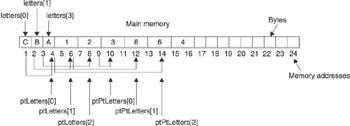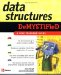# Chapter 3: What Is an Array?

Computer memory is like a small town ”or a large town, depending on the amount of memory available in the computer. Each byte of memory is a building that has its own address called a memory address , and the town s people are bits of data living in those buildings. The small town inside your computer is a neighborly place. A program refers to buildings by name rather than by address, and puts Mary s grade in the maryGrade building. However, being personable is troublesome when you need to come up with hundreds of names for these buildings. That is, unless you use an array. You ll explore arrays, multidimensional arrays, pointer arrays, and an array of pointers to pointers in this chapter.

## An Array

An array is a way to reference a series of memory locations using the same name. Each memory location is represented by an array element. An array element is similar to one variable except it is identified by an index value instead of a name. An index value is a number used to identify an array element.

Now we ll show you what an array looks like, with the three array elements shown next . The array is called grades . The first array element is called grades . The zero is the index value. The square bracket tells the computer that the value inside the square bracket is an index.

` grades grades grades `

Each array element is like a variable name. For example, the following variables are equivalent to array elements. There is no difference between array elements and variables ”well, almost no difference, but we ll get to the differences in a moment. For now, let s explore how they are the same. Here are three integer variables:

` int maryGrade; int bobGrade; int amberGrade; `

You probably recall from your programming class that you store a value into a memory location by using an assignment statement. Here are two assignment statements. The first assigns a value to a variable, and the other assigns a value to an array element. Notice that these statements are practically the same except reference is made to the index of the array element in the second statement:

` int grades; maryGrade = 90;  grades = 90; `

Suppose you want to use the value stored in a memory location. There are a number of ways to do this in a program, but a common way is to use another assignment statement like the ones shown in the next example. The first assignment statement uses two variables, the next assignment statement uses two array elements, and the last assignment statement assigns the value referenced by a variable name and assigns that value to an array element:

` bobGrade = maryGrade; grades = grades; grades = bobGrade; `

You ve probably noticed a pattern developing. You use an array element the same way you use a variable.

### Why an Array?

There are two important differences between an array element and a variable, and those differences make working with large amounts of data a breeze . Suppose you had to work with 100 grades to calculate the average grade. How would you do this?

The challenge isn t applying the formula for calculating an average. You know how that s done. The challenge is to come up with 100 variable names and then reference all those variable names in a program. Ouch!

First, you d need to sum all the grades by writing a statement similar to the following. (We ll stop at three variables because it s difficult to identify 100 variables ”and we d run out of space on this page.)

` sum = maryGrade + bobGrade + amberGrade; `

Now, here s how a smart programmer meets this challenge using an array:

` sum = 0; for (int i = 0; i < 100; i++)  sum = sum + grades[i]; `

Big difference. The control variable of the for loop is the index for the array element, enabling the program to quickly walk through all array elements in two lines of code. (The first statement has nothing to do with walking through all the array elements. It only initializes the sum variable with the total grades.)

The other difference between an array and a variable is that all the array elements are next to each other in memory. Variables can be anywhere in memory. For example, grades is next to grades in memory, grades is next to grades in memory, and so on. In contrast, maryGrade and bobGrade variables can be anywhere in memory, even if they are declared in the same declaration statement.

You might be scratching your head right now thinking, that s an interesting bit of computer trivia. So what? But the location of array elements is important when pointers (see Chapter 2) are used to manipulate data stored in memory. It is more efficient to point to array elements than variables because the computer moves to the next memory location when you point to the next array element.

### Arrays and Data Structures

Some programmers might say that arrays are the backbone of data structures because an array enables a programmer to easily reorganize hundreds of values stored in memory by using an array of pointers to pointers.

This is a mouthful to say. So we drew a picture to show you the importance of arrays in data structures. Figure 3-1 shows memory; you ll remember this from the previous chapters in this book. Each block is a byte. We ll say that two bytes are needed to store a memory address in memory. You need to store a memory address in memory because you ll use it to refer to other memory addresses in the An Array of Pointers section of this chapter.Figure 3-1: Elements of an array are stored sequentially in memory.

First, create an array called letters and assign characters to it, as shown here:

` char letters; letters = 'C'; letters = 'B'; letters = 'A'; `

You ll notice in Figure 3-1 that each letter appears one after the other in memory. This is because these values are assigned to elements of an array, and each array element is placed sequentially in memory.

Next, create an array of pointers. As you ll recall from Chapter 2, a pointer is a variable that contains a memory address of another variable. In this example, you ll use an array of pointers instead of a pointer variable.

An array of pointers is nearly identical to a pointer variable except each array element contains a memory address. Assign the memory address of each element of the letters array to elements of the ptLetters array, which is an array of pointers. Here s how this is done in C and C++:

` char * ptLetters; for (int i = 0; i < 3; i++)  ptLetters[i] = &letters[i]; `

Remember from Chapter 2 that the ampersand (&), which is called the address operator, tells the computer to assign the memory address of the element of the letters array and not the contents of the element.

The final step is to create an array of pointers to pointers and then use it to change the order of the letters array when printing the letters array on the screen. A pointer to a pointer is a variable that contains the address of another pointer. In the example, you use an array of pointers to a pointer where each element of the array is like a pointer to a pointer variable. That is, each element is assigned an address of a pointer.

Use the following code to assign the memory address of each element of the ptLetters pointer array to the ptPtLetters pointer to pointer array. Notice that you don t use a for loop. This is because you need to change the order of the letters array without changing the letters array itself. Figure 3-1 shows how memory looks after the following code executes. If you printed elements of the ptPtLetters array, what would be displayed on the screen?

` char ** ptPTLetters; ptPtLetters = &ptLetters; ptPtLetters = &ptLetters; ptPtLetters = &ptLetters; `

Here is the code that prints the ptPtLetters array:

` for (i = 0; i <3; i++)  cout << **ptPtLetters[i] << endl; `

The answer to the question is A B C . Follow Figure 3-1 as we explain how this works. The first element of the ptPtLetters array is located at memory address 10. The content of memory address 10 is 8, which is memory address 8 because memory address 10 is the last element of the array ptLetters ”a pointer. The value of memory address 8 is 3, which is the memory address of the third element of the array letters.

When the computer sees the ptPtLetters[i] statement for the first time, it goes to the array element ptPtLetters and reads its value, which is 8. The computer then goes to memory address 8 and reads its content because memory address 8 is a pointer. The content of memory address 8 is 3, which is the memory address of the third element of the letters array. The computer reads the content of memory address 3 and displays the content on the screen.

This can be a bit tricky to follow unless you use Figure 3-1 as a guide; you can also use Figure 3-1 to explain how the computer displays the other letters.

The importance of using arrays for data structures is that you can easily change the order of data by using pointers and pointers to pointers without having to touch the original data. Some smart programmer might tell you that you re not saving any time or memory by using pointers and pointers to pointers to rearrange an array of characters. The programmer is correct. However, we re juggling characters to illustrate how arrays and pointers to pointers work. In the real world, pointers typically point to a whole group of information such as a client s name, address, phone number, and other pertinent data. Instead of juggling all that information, you need only to juggle memory addresses.Data Structures Demystified (Demystified)
ISBN: 0072253592
EAN: 2147483647
Year: 2006
Pages: 90

Similar book on Amazon